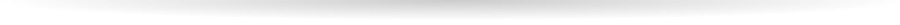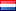Wiegerinck W.A.J.J., Kappen H.J., Burgers W.G.
Bayesian 网络构架的专家系统，理论和实际应用。

Bayesian Networks

Bayesian 网络系统非常适合于亲属间族系关系的基因组计算。 它能计算任何失踪人口和亲属关系。 而且Bayesian 网络系统的另外一个优势是非常透明和有弹性，允许你加入其他变量，如计算错误可能性，丢失数据，更先进的基因标记等等。

Bonaparte 软件的核心是使用了Bayesian 网络构架和交互手段，能够在DNA基础上，加上额外的族系关系，正确的做亲缘分析。这个软件对于处理大数据量的失踪人口和受害者的性能非常优越。

• 假说H0中p 我们假设MP=UI，在这样的情况下，MP是可以预见的而U是不可预见的。 E={DNAMP+DNAFAM}
• 假说H1 我们假设U=UI 无法验证身份的人，U是可以预见的和MP也是可以预见的。这个结果等于E = {DNAU +DNAFAM}

LR =P(E|Hp)/P(E|Hd)

P(Hp|E)/P(Hd|E)=( P(E|Hp)P(Hp) )/( P(E|Hd)P(Hd) )
DNA Profiles

(CATG)3=CATGCATGCATG

x̄ =(1x1;1x2);(2x1;2x2);⋯;(nx1;nx2)

DNA数据和家庭成员关系能够被族系谱构建，同时考虑遗传位点的转移。 在每个簇中，每个个人分别有一个父母染色体 （X或者f）。 成对的位点被翻译为 X。 xi =(xfi,xmi). 每个位点我们标记为 （X或m）。有时候我们有标注（S）,它的值可能是（f，m）因此每个簇在族谱系中能够被列为（i，m）。可以假设簇为N值。

P(xi|xf(i),xm(i)) = P(xfi|xf(i))P(xmi|xm(i))

P(xfi|xf(i)) = ½ Σs=f,m P(xfi|xsf(i))
P(xmi|xm(i)) = ½ Σs=f,m P(xmi|xsm(i))

P(a|a)=1-μ
P(a|b)= μ/(N-1)

P(x̄|y,L = 0 ) = 1 ⇔ x̄ = y, 0 otherwise.

P(x̄ = (a,F)|(a,b), L = 1) = ½ ⇔ a ≠ b
P(x̄ = (b,F)|(a,b, )L = 1) = ½ ⇔ a ≠ b

P(x̄=(a,F)|(a,a),L = 1) = 1

Inference

P( {x̄,x}J) = ∏j P(x̄j|xj,Lj) ∏i ∈ J\F P(xi|xf(i),xm(i)) ∏i ∈ F P(xi)

P({x̄}O) = Σx1 … ΣxKj ∈ O P(x̄j|xj,Lj) ∏ i ∈ J\FP(xi|xf(i),xm(i)) ∏i ∈F P(xi)

August 29 2014, 09:24:21 / 86b0d299abb5028bb2f79d36338db6fcaa956152
SMART Research BV • Heyendaalseweg 135 • 6525 AJ Nijmegen • The NetherlandsCookies